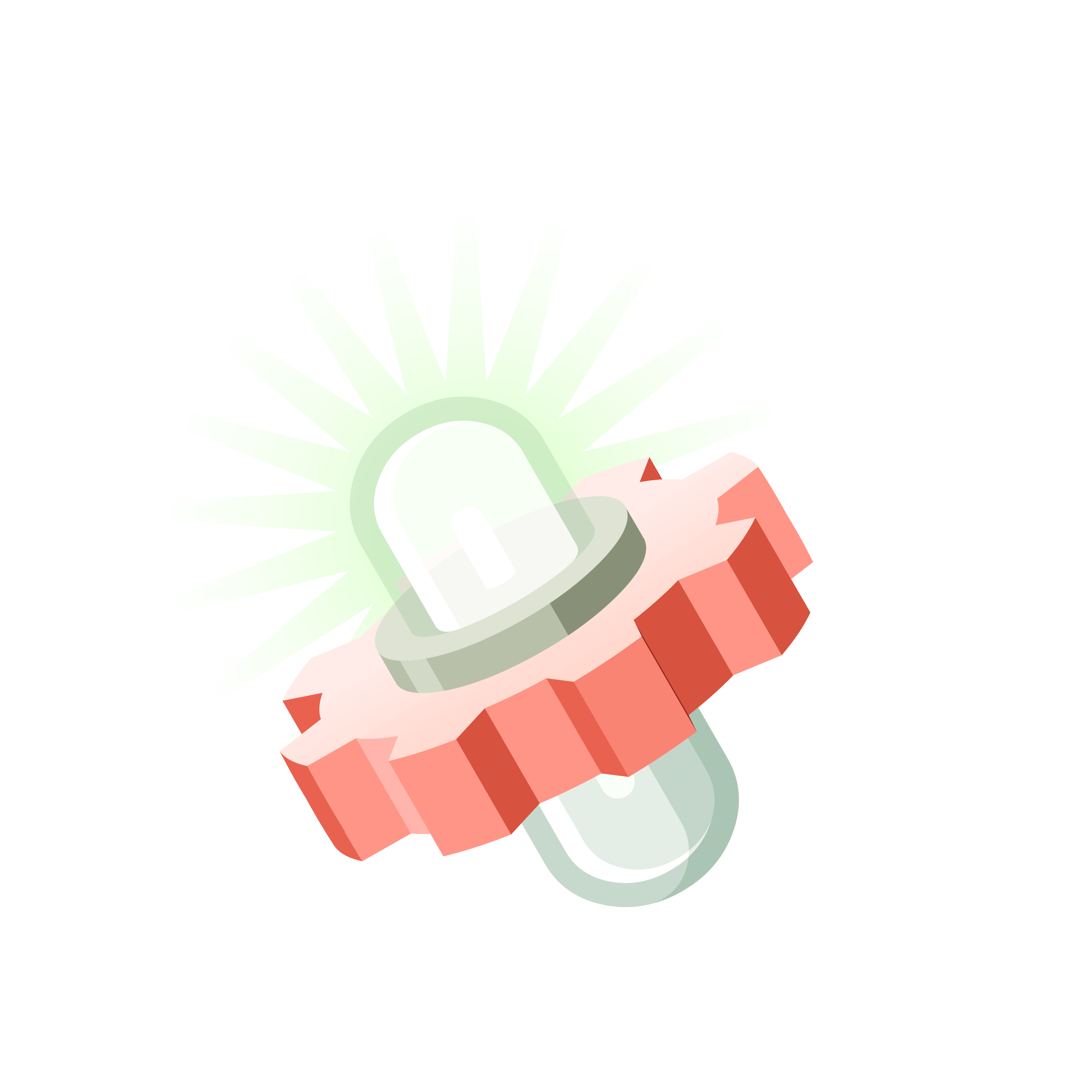05 217 142# Station  EWild ExplorationHere are some "big questions" for you to think about.
Have fun!

### Question 1

Jay decides to play with a machine that follows a $1 \leftarrow 1$ rule. He puts one dot into the rightmost box.
What happens? Do assume there are infinitely many boxes to the left.Suggi plays with a machine following the rule $2 \leftarrow 1$. She puts one dot into the right-most box. What happens in this case?

Do you think these machines are interesting? Is there much to study about them?

### Question 2

Poindexter decides to play with a machine that follows the rule $2 \leftarrow 3$.

Describe what happens when there are three dots in a box.### Question 3

Work out the $2\leftarrow 3$ machine codes for the numbers $1$ up to $30$. Any patterns?### Question 4

The code for ten in this machine turns out to be $2101$. Look at your code for twenty. Can you see it as the answer to "ten plus ten"? Does your code for thirty look like the answer to "ten plus ten plus ten"?Comment: We'll explore this weird $2\leftarrow 3$ machine later on.

### Let's Go Wild!